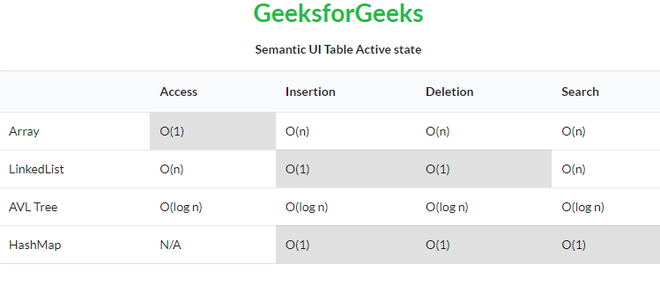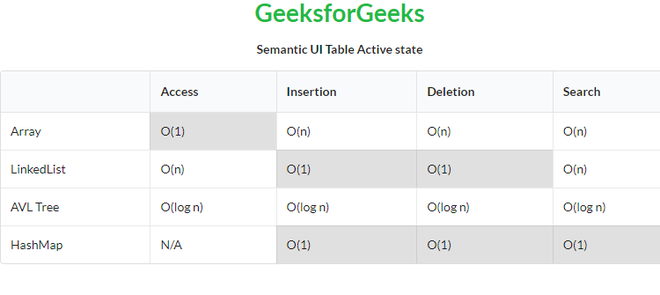GFG App
Open AppBrowser
Continue

# Semantic-UI Table Active State

Semantic UI is an open-source framework that uses CSS and jQuery to build great user interfaces. It is the same as a bootstrap for use and has great different elements to use to make your website look more amazing. It uses a class to add CSS to the elements.

Tables are an easy way to organize a lot of data. A table is an arrangement of data in rows and columns, or possibly in a more complex structure. Tables are widely used in communication, research, and data analysis. Tables are useful for various tasks such as presenting text information and numerical data. It can be used to compare two or more items in the tabular form layout. Tables are used to create databases. An HTML table and a Semantic UI table both are the same structurally.

Active state sets cell or row to be active or selected by a user.

Semantic UI Table Active state class:

• active: This class sets a cell or row to an active state.

Syntax:

```<table class="ui celled table">
<tr class="active">
<td></td>
...
</tr>
...
</table>```

Example 1: This is a basic example illustrating the table’s active state.

## HTML

 ` ` `<``html``> ` `<``head``> ` `    ``<``title``>Semantic UI Table Active state ` `    ``<``link` `href``= ` `"https://cdnjs.cloudflare.com/ajax/libs/semantic-ui/2.4.1/semantic.min.css"`  `         ``rel``=``"stylesheet"` `/> ` `    ``<``script` `src``= ` `"https://code.jquery.com/jquery-3.1.1.min.js"`  `          ``integrity``= ` `"sha256-hVVnYaiADRTO2PzUGmuLJr8BLUSjGIZsDYGmIJLv2b8="`  `          ``crossorigin``=``"anonymous"``>  ` `    `` ` `    ``<``script` `src``= ` `"https://cdnjs.cloudflare.com/ajax/libs/semantic-ui/2.4.1/semantic.min.js"``> ` `    `` ` ` ` `<``body``> ` `    ``<``center``> ` `        ``<``h1` `class``=``"ui green header"``>GeeksforGeeks ` `        ``<``strong``>Semantic UI Table Active state ` `    `` ` `        ``<``table` `class``=``"ui table"``> ` `            ``<``thead``> ` `              ``<``tr``><``th``> ` `              ``<``th``>Access ` `              ``<``th``>Insertion ` `              ``<``th``>Deletion ` `              ``<``th``>Search ` `            `` ` `            ``<``tbody``> ` `              ``<``tr``> ` `                ``<``td``>Array ` `                ``<``td` `class``=``"active"``>O(1) ` `                ``<``td``>O(n) ` `                ``<``td``>O(n) ` `                ``<``td``>O(n) ` `              `` ` `              ``<``tr``> ` `                ``<``td``>LinkedList ` `                ``<``td``>O(n) ` `                ``<``td` `class``=``"active"``>O(1) ` `                ``<``td` `class``=``"active"``>O(1) ` `                ``<``td``>O(n) ` `              `` ` `              ``<``tr``> ` `                ``<``td``>AVL Tree ` `                ``<``td``>O(log n) ` `                ``<``td``>O(log n) ` `                ``<``td``>O(log n) ` `                ``<``td``>O(log n) ` `              `` ` `              ``<``tr``> ` `                ``<``td``>HashMap ` `                ``<``td``>N/A ` `                ``<``td` `class``=``"active"``>O(1) ` `                ``<``td` `class``=``"active"``>O(1) ` `                ``<``td` `class``=``"active"``>O(1) ` `              `` ` `          `` ` `        `` ` ` `` ` ``

Output:Example 2: This is a basic example illustrating the celled table active state.

## HTML

 ` ` `<``html``> ` `<``head``> ` `        ``<``title``>Semantic UI Table Active state ` `        ``<``link` `href``=  ` `"https://cdnjs.cloudflare.com/ajax/libs/semantic-ui/2.4.1/semantic.min.css"`  `             ``rel``=``"stylesheet"` `/> ` `        ``<``script` `src``= ` `"https://code.jquery.com/jquery-3.1.1.min.js"`  `              ``integrity``= ` `"sha256-hVVnYaiADRTO2PzUGmuLJr8BLUSjGIZsDYGmIJLv2b8="` `crossorigin``=``"anonymous"``>  ` `       `` ` `        ``<``script` `src``= ` `"https://cdnjs.cloudflare.com/ajax/libs/semantic-ui/2.4.1/semantic.min.js"``> ` `        `` ` ` ` `<``body``> ` `        ``<``center``> ` `            ``<``h1` `class``=``"ui green header"``>GeeksforGeeks ` `            ``<``strong``>Semantic UI Table Active state ` `        `` ` `        ``<``table` `class``=``"ui table celled"``> ` `            ``<``thead``> ` `              ``<``tr``><``th``> ` `              ``<``th``>Access ` `              ``<``th``>Insertion ` `              ``<``th``>Deletion ` `              ``<``th``>Search ` `            `` ` `            ``<``tbody``> ` `              ``<``tr``> ` `                ``<``td``>Array ` `                ``<``td` `class``=``"active"``>O(1) ` `                ``<``td``>O(n) ` `                ``<``td``>O(n) ` `                ``<``td``>O(n) ` `              `` ` `              ``<``tr``> ` `                ``<``td``>LinkedList ` `                ``<``td``>O(n) ` `                ``<``td` `class``=``"active"``>O(1) ` `                ``<``td` `class``=``"active"``>O(1) ` `                ``<``td``>O(n) ` `              `` ` `              ``<``tr``> ` `                ``<``td``>AVL Tree ` `                ``<``td``>O(log n) ` `                ``<``td``>O(log n) ` `                ``<``td``>O(log n) ` `                ``<``td``>O(log n) ` `              `` ` `              ``<``tr``> ` `                ``<``td``>HashMap ` `                ``<``td``>N/A ` `                ``<``td` `class``=``"active"``>O(1) ` `                ``<``td` `class``=``"active"``>O(1) ` `                ``<``td` `class``=``"active"``>O(1) ` `              `` ` `          `` ` `        `` ` `    `` ` ``

Output:My Personal Notes arrow_drop_up